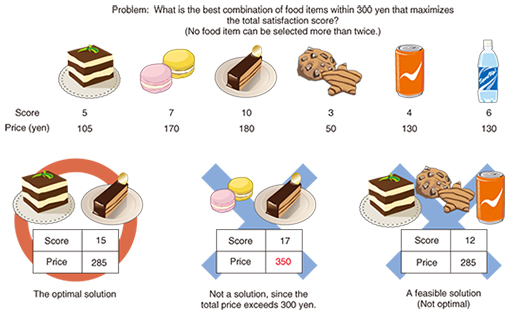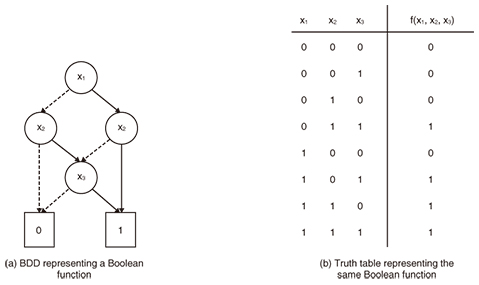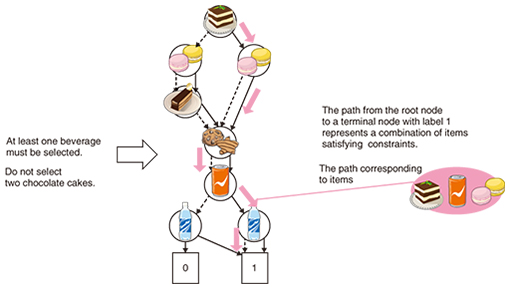Feature Articles: Communication Science as a Compass for the Future

# Combinatorial Optimization Using Binary Decision Diagrams

## Abstract

Combinatorial optimization is being used to solve a wide range of real world tasks, but its application requires that we formulate the task as an optimization problem for which efficient methods for solving the problem exist. However, sometimes task-specific constraints prevent us from formulating the task as an easy-to-solve optimization problem. In this article, we present a new algorithm for solving combinatorial optimization problems by using a binary decision diagram (BDD), a data structure for representing a Boolean function as a compact graph. Our method can efficiently solve constraint-added variants of a class of optimization problems by representing the constraints with a BDD or zero-suppressed BDD (ZDD) and then applying an efficient dynamic programming algorithm.

Keywords: combinatorial optimization, binary decision diagram, natural language processing

## 1. Introduction

In daily life, we make many decisions ranging from important decisions on business matters to choosing what to eat for lunch. How do people choose one action from all possible actions? It seems natural to assume that people rely on some criteria for selecting their action, rather than selecting it at random. Here, we set the assumption that every possible action can be scored, a value that represents how good that action is. Under this assumption, making a decision can be regarded as solving the problem of finding an action with a maximum score. This is called an optimization problem. If all possible candidates are represented as the assignment of discrete values on variables, then the problem is called a combinatorial optimization problem. In addition to decision making in daily life, techniques that involve solving combinatorial optimization problems are used in performing various computer science tasks.

## 2. Solving real world tasks with combinatorial optimization techniques

To solve a real world task using combinatorial optimization, we first have to formulate the task as an optimization problem, and then solve the problem. The formulation consists of (i) setting constraints that all possible actions must satisfy and (ii) designing an objective function that takes a possible choice and returns the score that represents how good the choice is. Let the choices satisfying the constraints be possible solutions, and let the possible solution that maximizes the objective function be the optimal solution.

We can solve several tasks by formulating them as combinatorial optimization problems. As a very simple example, let us consider the situation in which someone wants to buy some food at a grocery store under the constraint that the total price spent must be within 300 yen. We assume that the store sells n kinds of items, and every item has a satisfaction score. The score represents the degree to which the user will be satisfied upon buying the item(s). Under this assumption, the task of buying food is formulated as the combinatorial optimization problem of finding the best combination that maximizes the sum of satisfaction scores while keeping the total price within 300 yen (Fig. 1). We can get the best combination of items within 300 yen by solving the optimization problem.Fig. 1. A combinatorial optimization problem.

The degree of difficulty in solving an optimization problem depends on the problem. Thus, it is important to formulate a task as a combinatorial optimization problem for which efficient solution algorithms exist. The knapsack problem and the shortest path problem are typical examples of combinatorial optimization problems, and their algorithms are often applied. Unfortunately, such algorithms fail to offer full problem coverage due to certain limitations. For example, the above example of buying items can be formulated as a knapsack problem, and we can find the optimal solution in linear time (n × L, where n is the number of items and L is the budget). However, the knapsack algorithm fails to consider relationships between selected items. This means that conditions such as should not buy item A and item B at the same time, or should buy at least item C or D cannot be considered when finding the optimal solution.

Many task-specific conditions must be considered when solving real world tasks, and these constraints prevent the tasks from being formulated as easy-to-solve combinatorial optimization problems. If no efficient algorithm exists for solving a problem, we have two choices; the first is to develop a new solution algorithm, and the second is to use a general-purpose optimization software package. Taking the former approach is unrealistic because it is virtually impossible for non-experts to design an efficient algorithm. Taking the latter approach is relatively easy, and off-the-shelf software can be used to solve various kinds of optimization problems. However, this approach has a shortcoming in that we cannot estimate the time required for solving the problem beforehand.

We have developed a new efficient optimization algorithm for solving a class of optimization problems. This class consists of problems that can be formulated as the addition of constraints that consider discrete relationships among variables to easy-to-solve optimization problems such as the knapsack problem. The main feature of our method is its use of a binary decision diagram (BDD) or zero-suppressed BDD (ZDD) to represent the additional constraints. BDDs and ZDDs are data structures that represent a Boolean function as a compact graph. We first cast the additional constraints as a BDD or ZDD, then subject the resulting structure to a dynamic programming algorithm to solve the constraint-added problem in time linear to the number of BDD or ZDD nodes.

## 3. Binary decision diagrams (BDDs, ZDDs)

BDDs and ZDDs are data structures that represent a Boolean function as a graph. A Boolean function takes n input binary variables (variables that take either 0 or 1) and returns either 0 or 1. A BDD represents a Boolean function as a graph, as shown in Fig. 2(a). There are several ways to represent a Boolean function other than with a BDD, such as a truth table (Fig. 2(b)) or a binary decision tree. The BDD differs from these representations in that it can represent an n-ary Boolean function as a BDD whose number of nodes is much smaller than 2n; other representations require 2n elements to represent the same Boolean function. The BDD also supports several operations that run in time proportional to the number of BDD nodes, and two BDDs can be subjected to Boolean operations to efficiently create another BDD.Fig. 2. Representing a Boolean function using a binary decision diagram.

A ZDD is a variant of a BDD and also represents a Boolean function as a graph. The ZDD differs from the BDD in that it can represent a family of sets as a DAG (directed acyclic graph) with fewer nodes than a BDD.

## 4. Combinatorial optimization using BDD and ZDD

We previously proposed some optimization algorithms that use BDDs and ZDDs [1, 2]. In this article, we discuss an algorithm that uses a ZDD to solve constraint-added variants of the 0-1 knapsack problem. The 0-1 knapsack problem is an optimization problem in which we are given n items that have their own costs and scores, and we must find the best subset of items that maximizes the sum of scores while keeping the sum of costs within the given threshold. A 0-1 knapsack problem can be solved efficiently by applying a dynamic programming algorithm.

Our algorithm can solve the problem made by adding constraints between variables to a 0-1 knapsack problem. As shown above, there is no efficient dynamic programming algorithm for ordinary 0-1 knapsack problems that contain additional constraints. However, if we represent such constraints by a ZDD, we can apply a dynamic programming algorithm that exploits the structure of the ZDD to solve constraint-added knapsack problems (Fig. 3). Our algorithm can solve a problem in O (Z × L) time, where Z is the number of ZDD nodes, and L is the threshold of total costs. We can efficiently solve such problems if the added constraints can be represented by a small ZDD.Fig. 3. Representation of constraints using ZDD.

## 5. Application to natural language processing

We applied our algorithm to text summarization, a common natural language processing task . Text summarization is the task of making a concise summary of an input document. Many text summarization approaches have been proposed, and one of the most popular approaches is to extract important sentences from the input document. Our research group proposed an extraction-based summarization method that first casts the input as a dependency structure tree that represents the dependency between clauses and then makes a summary by finding the optimal subtree . Since this summarization method considers the dependency between clauses in making a summary, it can generate consistent summaries. Unfortunately, no efficient algorithm for solving this optimization problem has been developed until now.

This problem of finding the optimal subtree can be regarded as a 0-1 knapsack problem with the constraint that a solution must be a subtree of the input tree. This is a discrete constraint imposed on the relation between variables, so we represent it as a ZDD and solve the problem with our ZDD-based optimization method, which can solve problems up to 300 times faster than previous approaches. Furthermore, we prove that the number of ZDD nodes in the set of all subtrees of an input tree is bounded to n log n, where n is the number of nodes of the input tree. This result suggests that our method can be applied to large problems.

## 6. Future work

Since combinatorial optimization is relevant to many real world tasks, we believe our method can be used in fields other than natural language processing. We will continue to improve and analyze the performance of our algorithm in order to improve its effectiveness and efficiency.

## References

 M. Nishino, N. Yasuda, S. Minato, and M. Nagata, “BDD-constrained Search: A Unified Approach to Constrained Shortest Path Problems,” Proc. of AAAI-15 (Twenty-Ninth AAAI Conference on Artificial Intelligence), pp. 1219–1225, Austin, USA, Jan. 2015. M. Nishino, N. Yasuda, T. Hirao, S. Minato, and M. Nagata, “A Dynamic Programming Algorithm for Tree Trimming-based Text Summarization,” Proc. of NAACL-HLT 2015 (2015 Annual Conference of the North American Chapter of the Association for Computational Linguistics: Human Language Technologies), pp. 462–471, Denver, USA, June 2015. T. Hirao, Y. Yoshida, M. Nishino, N. Yasuda, and M. Nagata, “Single-document Summarization as a Tree Knapsack Problem,” Proc. of EMNLP 2013 (2013 Conference on Empirical Methods in Natural Language Processing), pp. 1515–1520, Seattle, USA, Oct. 2013.Masaaki Nishino Researcher, NTT Communication Science Laboratories. He received his B.E., M.E., and Ph.D. in informatics from Kyoto University in 2006, 2008, and 2014. He joined NTT in 2008. His current research interests include natural language processing and combinatorial optimization. Norihito Yasuda Research Associate Professor, Graduate School of Information Science and Technology, Hokkaido University. He received a B.A. in integrated human studies and an M.A. in human and environmental studies from Kyoto University in 1997 and 1999, and a D.Eng. in computational intelligence and system science from Tokyo Institute of Technology in 2011. He joined NTT in 1999. He is currently also a Research Associate Professor with the Graduate School of Information Science and Technology, Hokkaido University. His current research interests include discrete algorithms and natural language processing. Tsutomu Hirao Senior Research Scientist, NTT Communication Science Laboratories. He received a B.E. from Kansai University in 1995, and an M.E. and Ph.D. in engineering from Nara Institute of Science and Technology in 1997 and 2002. His current research interests include natural language processing and machine learning. Shin-ichi Minato Professor, Graduate School of Information Science and Technology, Hokkaido University. He received his B.E., M.E., and D.E. in information science from Kyoto University in 1988, 1990, and 1995. He worked for NTT from 1990 until 2004. He was a Visiting Scholar in the Computer Science Department of Stanford University in 1997. He joined Hokkaido University as an Associate Professor in 2004 and has been a full Professor since October 2010. He has served as the Research Director of the ERATO MINATO Discrete Structure Manipulation System Project, executed by the Japan Science and Technology Agency, since 2009. His research topics include efficient representations and manipulation algorithms for large-scale discrete structure data. He published “Binary Decision Diagrams and Applications for VLSI CAD” (Kluwer, 1995). He is a senior member of the Institute of Electronics, Information and Communication Engineers (IEICE) and the Information Processing Society of Japan (IPSJ), and a member of the Institute of Electrical and Electronics Engineers, and the Japanese Society for Artificial Intelligence (JSAI). Masaaki Nagata Senior Distinguished Researcher, Group Leader, NTT Communication Science Laboratories. He received his B.E., M.E., and Ph.D. in information science from Kyoto University in 1985, 1987, and 1999. He joined NTT in 1987. He was with ATR Interpreting Telephony Research Laboratories from 1989 to 1993 and was a Visiting Researcher at AT&T Laboratories Research, New Jersey, USA, from 1999 to 2000. His research interests include natural language processing, especially morphological analysis, named entity recognition, parsing, and machine translation. He is a member of IEICE, IPSJ, JSAI, the Association for Natural Language Processing, and the Association for Computational Linguistics.

↑ TOP